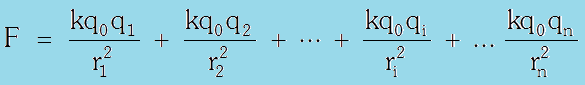# Principle of superposition

Principle of superposition :- The force is a two body interaction, i.e., electrical force between two point charges is independent of presence or absence of other charges and so the principle of superposition is valid, i.e.,

force on a charged particle due to number of point charges is the resultant of forces due to individual point charges”When a number of charges are interacting, the total force on a given charge is vector sum of the forces exerted on it by all other charges individuallySample problems with solution:-

Example (A)
Three equal point charges of charge +q are moving along a circle of radius R and a point charge –2q is also placed at the Centre of circle as (shown in figure), if charges are revolving with constant and same
speed then calculate speed.

Example (B)
Two equally charged identical small metallic spheres A and B repel each other with a force 2 × 10–5N when placed in air (neglect gravitation attraction). Another identical uncharged sphere C is touched to B and then placed at the mid point of line joining A and B. What is the net electrostatic force on C?

Example (C)
Five point charges, each of value q are placed on five vertices of a regular hexagon of side L. What is the magnitude of the force on a point charge of value – q coulomb placed at the centre of the hexagon?

Solutions:-

Superposition num